Next: Fresnel Relations Up: Multi-Dimensional Waves Previous: Polarization of Electromagnetic Waves

# Laws of Geometric Optics

Suppose that the region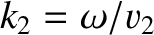is occupied by a transparent dielectric medium of refractive index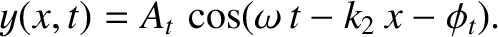, whereas the region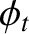is occupied by a second transparent dielectric medium of refractive index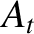. (See Figure 44.) Let a plane light wave be launched toward positivefrom a light source of angular frequency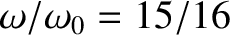located at large negative. Furthermore, suppose that this wave, which has the wavevector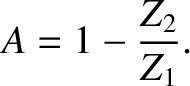, is obliquely incident on the interface between the two media. We would expect the incident plane wave to be partially reflected and partially refracted (i.e., transmitted) by the interface. (See Section 7.7.) Let the reflected and refracted plane waves have the wavevectors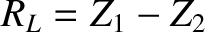and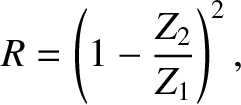, respectively. (See Figure 44.) Hence, we can write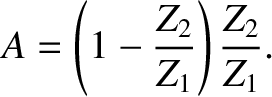(568)

in the region, and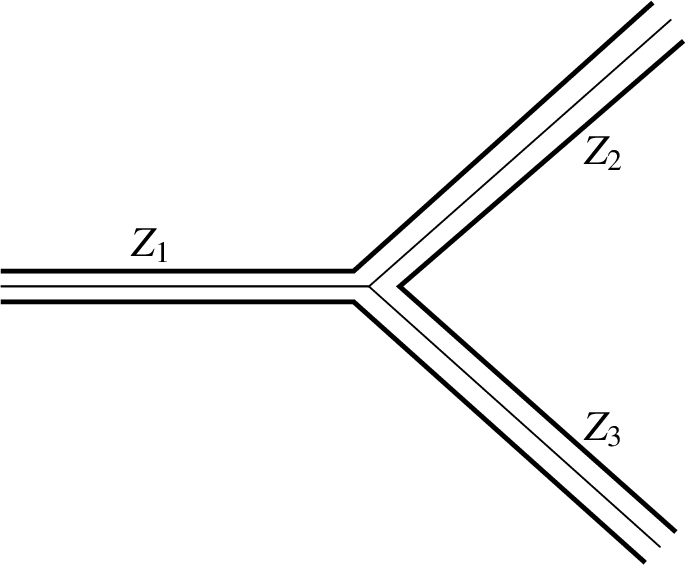(569)

in the region. Here,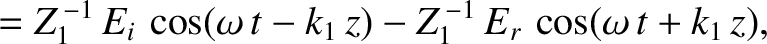represents the magnetic component of the resultant light wave,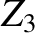the amplitude of the incident wave,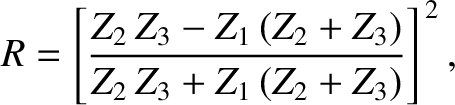the amplitude of the reflected wave, and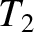the amplitude of the refracted wave. All of the component waves have the same angular frequency,, because this property is ultimately determined by the wave source. Furthermore, according to standard electromagnetic theory (Fitzpatrick 2008), if the magnetic component of an electromagnetic wave is specified then the electric component of the wave is fully determined, and vice versa.

In general, the wavefunction,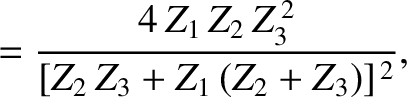, must be continuous at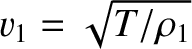, because, according to standard electromagnetic theory (see Appendix C), there cannot be a discontinuity in either the normal or the tangential component of a magnetic field across an interface between two (non-magnetic) dielectric media. [The same is not true of an electric field, which can have a normal discontinuity across an interface between two dielectric media (ibid.). (See Section 8.8.) This explains why we have chosento represent the magnetic, rather than the electric, component of the wave.] Thus, the matching condition attakes the form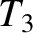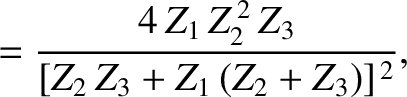(570)

This condition must be satisfied at all values of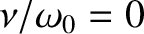,, and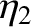. This is only possible if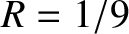(571)

and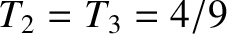(572)

Suppose that the direction of propagation of the incident wave lies in the-plane, so that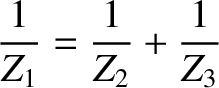. It immediately follows, from Equation (572), that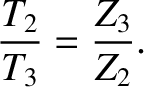. In other words, the directions of propagation of the reflected and the refracted waves also lie in the-plane, which implies that,andare co-planar vectors. This constraint is implicit in the well-known laws of geometric optics (Hecht 1974).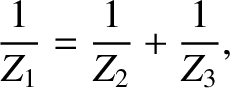Assuming that the previously mentioned constraint is satisfied, let the incident, reflected, and refracted wave normals subtend angles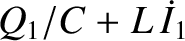,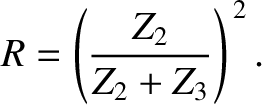, and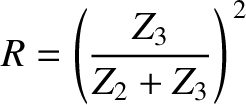with the-axis, respectively. (See Figure 44.) It follows that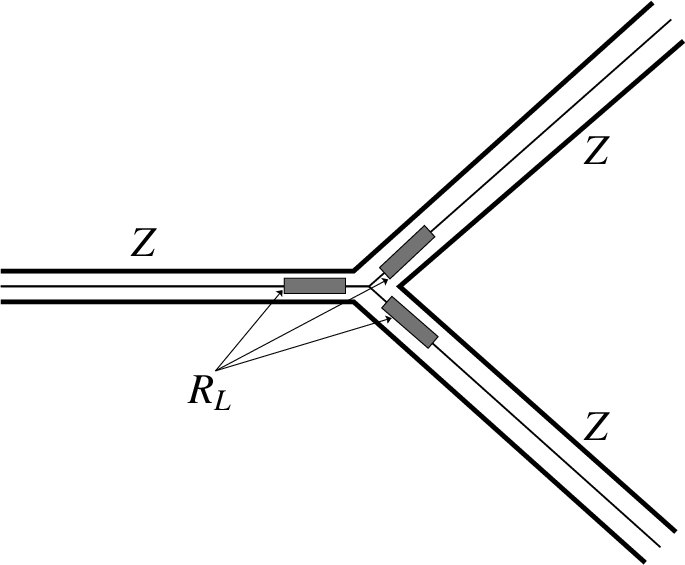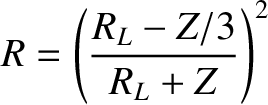(573)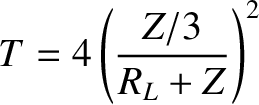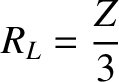(574)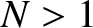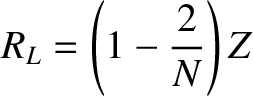(575)

where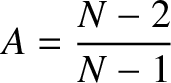is the vacuum wavenumber, and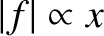the velocity of light in vacuum. Here, we have made use of the fact that wavenumber (i.e., the magnitude of the wavevector) of a light wave propagating through a dielectric medium of refractive index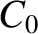is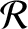. (See Section 7.7.)

According to Equation (571),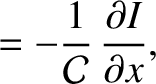, which yields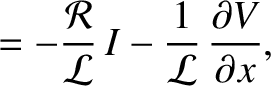(576)

and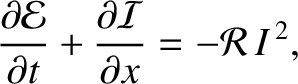, which reduces to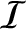(577)

The first of these relations states that the angle of incidence,, is equal to the angle of reflection,. This is the familiar law of reflection (Hecht 1974). Furthermore, the second relation corresponds to the equally familiar law of refraction, otherwise known as Snell's law (ibid.).

Incidentally, the fact that a plane wave propagates through a uniform dielectric medium with a constant wavevector, and, therefore, a constant direction of motion, is equivalent to the well-known law of rectilinear propagation, which states that a light ray (i.e., the normal to a constant phase surface) propagates through a uniform medium in a straight-line (Hecht 1974).

It follows, from the previous discussion, that the laws of geometric optics (i.e., the law of rectilinear propagation, the law of reflection, and the law of refraction) are fully consistent with the wave properties of light, despite the fact that they do not seem to explicitly depend on these properties.Next: Fresnel Relations Up: Multi-Dimensional Waves Previous: Polarization of Electromagnetic Waves
Richard Fitzpatrick 2013-04-08